A particle of charge q and mass m moves in a circular orbit of radius r with angular speed $\omega$ . The ratio of the magnitude of its magnetic moment to that of its angular momentum depends on

(a)                         (b)

(c)                         (d)

Concept Questions :-

Magnetic moment
High Yielding Test Series + Question Bank - NEET 2020

Difficulty Level:

An elastic circular wire of length L carries a current I. It is placed in a uniform magnetic field $\stackrel{\to }{B}$ (out of paper) such that its plane is perpendicular to the direction of $\stackrel{\to }{B}$ . The wire will experience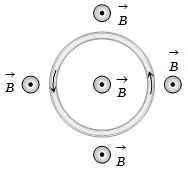(a) No force                            (b) A stretching force

(c) A compressive force            (d) A torque

Concept Questions :-

Current carrying loop : Force and torque
High Yielding Test Series + Question Bank - NEET 2020

Difficulty Level:

Wires 1 and 2 carrying currents ${i}_{1}$ and ${i}_{2}$ respectively are inclined at an angle $\theta$ to each other. What is the force on a small element dl of wire 2 at a distance of r from wire 1 (as shown in figure) due to the magnetic field of wire 1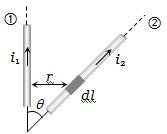(a)                               (b)

(c)                                (d)

Concept Questions :-

Force between current carrying wires
High Yielding Test Series + Question Bank - NEET 2020

Difficulty Level:

A conducting loop carrying a current I is placed in a uniform magnetic field pointing into the plane of the paper as shown. The loop will have a tendency to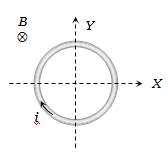(a) Contract                                        (b) Expand

(c) Move towards +ve x -axis               (d) Move towards -ve x -axis

Concept Questions :-

Current carrying loop : Force and torque
High Yielding Test Series + Question Bank - NEET 2020

Difficulty Level:

A current-carrying loop is placed in a uniform magnetic field in four different orientations, I, II, III & IV. The decreasing order of potential energy is :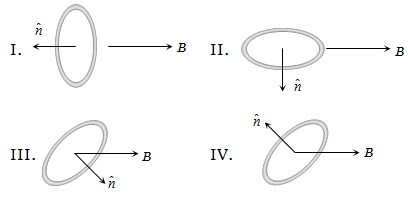(a) I > III > II > IV                               (b) I > II >III > IV
(c) I > IV > II > III                               (d) III > IV > I > II

Concept Questions :-

Current carrying loop : Force and torque
High Yielding Test Series + Question Bank - NEET 2020

Difficulty Level:

A metallic block carrying current I is subjected to a uniform magnetic induction $\stackrel{⇁}{B}$ as shown in the figure. The moving charges experience a force given $\stackrel{⇁}{F}$ by ........... which results in the lowering of the potential of the face ........ Assume the speed of the carriers to be v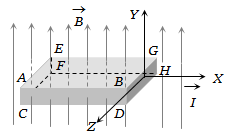(a) $eVB\stackrel{^}{k}$ , ABCD                                (b) $eVB\stackrel{^}{k}$ , EFGH

(c) $-eVB\stackrel{^}{k}$ , ABCD                              (d) $-eVB\stackrel{^}{k}$ , EFGH

Concept Questions :-

Lorentz force
High Yielding Test Series + Question Bank - NEET 2020

Difficulty Level:

Two insulated rings, one of slightly smaller diameter than the other are suspended along their common diameter as shown. Initially the planes of the rings are mutually perpendicular. When a steady current is set up in each of them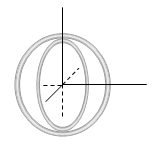(a) The two rings rotate into a common plane

(b) The inner ring oscillates about its initial position

(c) The inner ring stays stationary while the outer one moves into the plane of the inner ring

(d) The outer ring stays stationary while the inner one moves into the plane of the outer ring

Concept Questions :-

Magnetic field due to various cases

Difficulty Level:

Two particles each of mass m and charge q are attached to the two ends of a light rigid rod of length 2R. The rod is rotated at constant angular speed about a perpendicular axis passing through its centre. The ratio of the magnitudes of the magnetic moment of the system and its angular momentum about the centre of the rod is:

1. $\frac{q}{2m}$

2. $\frac{q}{m}$

3. $\frac{2q}{m}$

4. $\frac{q}{\mathrm{\pi m}}$

Concept Questions :-

Magnetic moment
High Yielding Test Series + Question Bank - NEET 2020

Difficulty Level:

A ring of radius R, made of an insulating material carries a charge Q uniformly distributed on it. If the ring rotates about the axis passing through its center and normal to the plane of the ring with constant angular speed $\omega$, then the magnitude of the magnetic moment of the ring is:

1. $Q\omega {R}^{2}$                       2. $\frac{1}{2}Q\omega {R}^{2}$

3. $Q{\omega }^{2}R$                       4. $\frac{1}{2}Q{\omega }^{2}R$

Concept Questions :-

Magnetic moment
High Yielding Test Series + Question Bank - NEET 2020

Difficulty Level:

Three long, straight and parallel wires carrying currents are arranged as shown in the figure. The wire C which carries a current of 5.0 amp is so placed that it experiences no force. The distance of wire C from wire D is then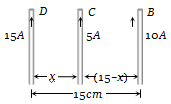(a) 9 cm                                          (b) 7 cm

(c) 5 cm                                           (d) 3 cm

Concept Questions :-

Force between current carrying wires